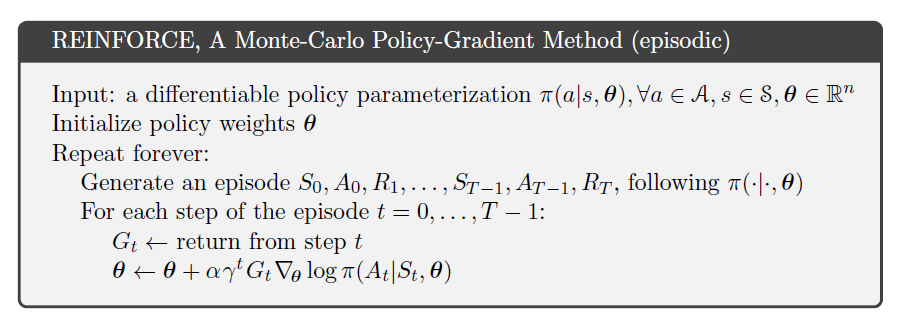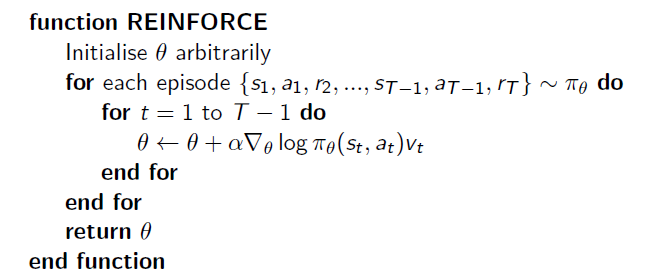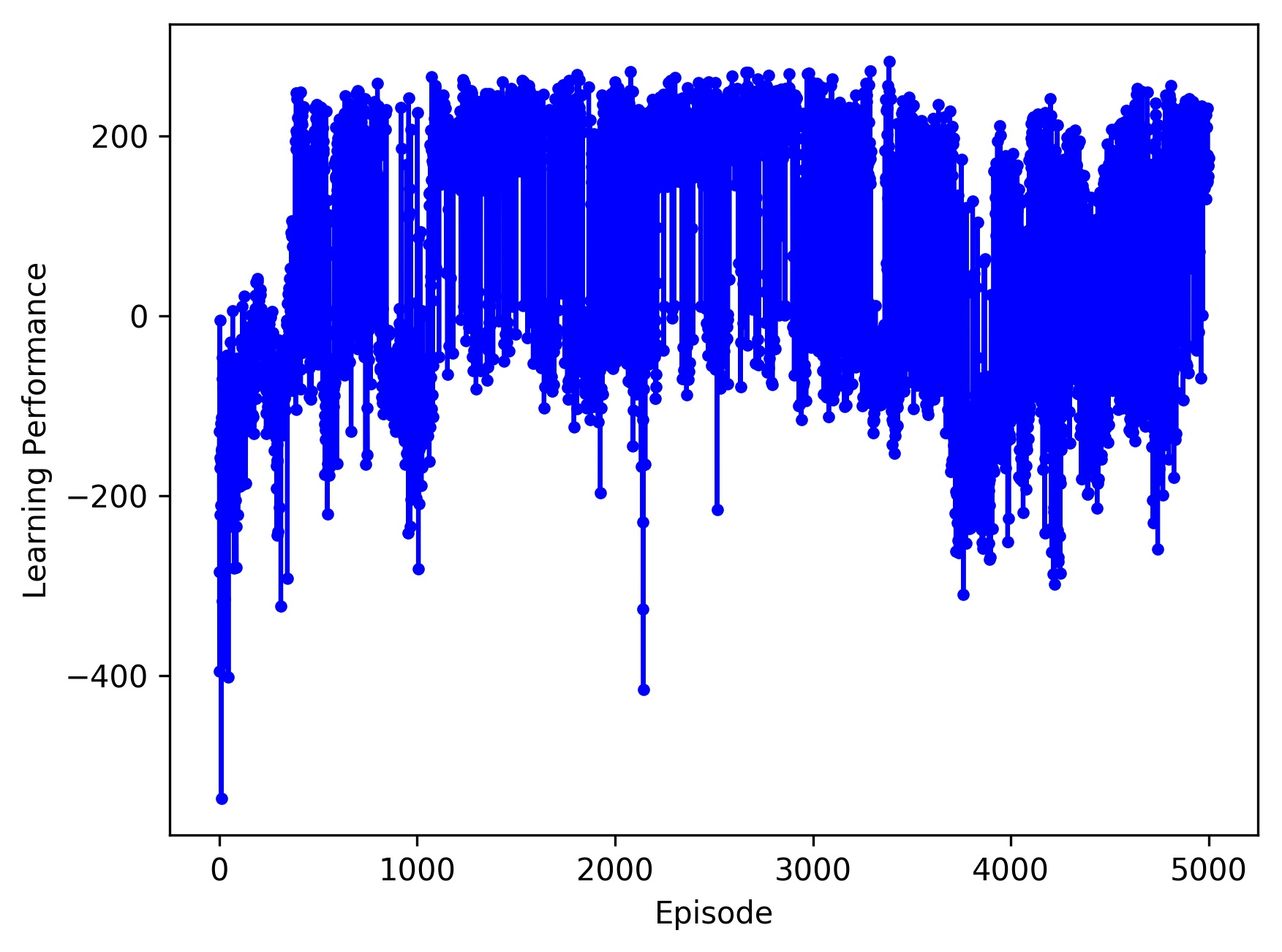### Lei Mao

Machine Learning, Artificial Intelligence, Computer Science.

# Monte Carlo Policy Gradient in OpenAI-Gym LunarLander

### Introduction

LunarLander is one of the learning environments in OpenAI Gym. I have actually tried to solve this learning problem using Deep Q-Learning which I have successfully used to train the CartPole environment in OpenAI Gym and the Flappy Bird game. However, I was not able to get good training performance in a reasonable amount of episodes. The lunarlander controlled by AI only learned how to steadily float in the air but was not able to successfully land within the time requested.

Here I am going to tackle this LunarLander problem using a new algorithm called “REINFORCE” or “Monte Carlo Policy Gradient”.### Touch the Algorithm

The algorithm from Sutton Book draftThe algorithm from Silver CoursewareNote that the $G_t$ item in Sutton’s REINFORCE algorithm and the $v_t$ item in Silver’s REINFORCE algorithm are the same things.

$G_t = R_{t+1} + \gamma \times R_{t+2} + \gamma^2 \times R_{t+3} + ... + \gamma^{T-t+1} \times R_{T}$

However, Silver’s REINFORCE algorithm lacked a $\gamma^t$ item than Sutton’s algorithm. It turned out that both of the algorithms are correct. Sutton’s algorithm worked for the episodic case maximizing the value of start state, while Silver’s algorithm worked for the continuing case maximizing the averaged value. The lunarlander problem is a continuing case, so I am going to implement Silver’s REINFORCE algorithm without including the $\gamma^t$ item.

### Make OpenAI Deep REINFORCE Class

The main neural network in Deep REINFORCE Class, which is called the policy network, takes the observation as input and outputs the softmax probability for all actions available.

This algorithm is very conceptually simple. However, I got stuck for a while when I first tried to implement it on my computer. We have got used to using deep learning libraries, such as TensorFlow, to calculate derivatives for convenience. The TensorFlow allows us to optimize the parameters in the neural network by minimizing some loss functions. However, from the REINFORCE algorithm, it seems that we have to manually calculate the derivatives and optimize the parameters through iterations.

One of way to overcome this is to construct a loss function whose minimization derivative udpate is exactly the same as the one in the algorithm. One simple loss function could be $-\log{\pi}(A_t \mid S_t,\theta) \times v_t$. Note that $-\log{\pi}(A_t \mid S_t,\theta)$ is the cross entropy of softmaxed action prediction and labeled action.

### Test OpenAI Deep REINFORCE Class in OpenAI Gym LunarLander Environment

#### Key Parameters

FC-16 -> FC-32

GAMMA = 0.99 # decay rate of past observations
LEARNING_RATE = 0.005 # learning rate in deep learning
RAND_SEED = 0 # random seed


#### Algorithm Performance

Before Training:After Training:#### OpenAI Gym Evaluation

Solved after 1476 episodes. The best 100-episode average reward was 203.29 ± 4.98.

https://gym.openai.com/evaluations/eval_6QdRxa5TuOD6GbmpbpsCw

This algorithm did solve the problem as OpenAI Gym requested. However, it suffered from a high variance problem. I tried to tune the hyperparameters and change the size of the neural network. But this did not help significantly.https://github.com/leimao/OpenAI_Gym_AI/tree/master/LunarLander-v2/REINFORCE/2017-05-24-v1

### Conclusions

REINFORCE Monte Carlo Policy Gradient solved the LunarLander problem which Deep Q-Learning did not solve. However, it suffered from a high variance problem. One may try REINFORCE with baseline Policy Gradient or actor-critic method to reduce variance during the training. I will write a blog once I implemented these new algorithms to solve the LunarLander problem.

#### 2017-5-4

To implement Policy Gradients Reinforcement Learning, I recommended using Tensorflow but not Keras, because you may have to introduce a lot of user-defined loss functions. Some of the customized loss functions could be easily defined in Keras, some of them are not. If you are comfortable with doing gradient descent by yourself, you do not even have to use TensorFlow.

I also tried REINFORCE to solve CartPole and MountainCar Problem in OpenAI Gym.

REINFORCE successfully solved CartPole in a very short period of time. However, it still suffered from a high variance problem (example). After tuning the model, one may get reasonable learning performance without too much variance(example). The code example could be found here.

REINFORCE never solved MountainCar problem unless I cheated. This is because it is extremely difficult (probability is extremely low) to get the top of the mountain without learning thoroughly. The learning agent always gets -200 reward in each episode. Therefore, the learning algorithm is useless. However, if the MountainCar problem is unwrapped, which means the game lasts forever unless the car goes to the top of the mountain, there could be appropriate gradient descent to solve the problem. Alternatively, one could engineer the reward that the API returns. By rewarding differently, say the higher the car goes the more reward is received, the car could easily learn how to climb. However, these are considered cheating because these do not provide any proof of the goodness of the learning algorithm itself.